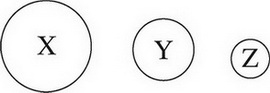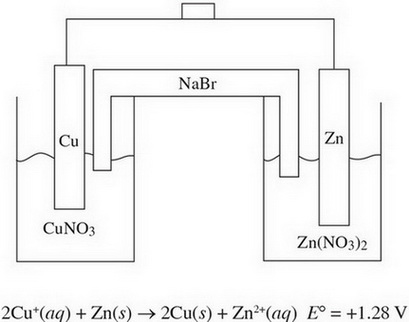# AP Chemistry Practice Test 1

### Test Information12 questions18 minutes

1. 2ClF(g) + O2(g) ↔ Cl2O(g) + F2O(g) &DELTA;H = 167 kJ/molrxn

During the reaction above, the product yield can be increased by increasing the temperature of the reaction. Why is this effective?

2. CH3NH2(aq) + H2O(l) ↔ OH-(aq) + CH3NH3+(aq)

The above equation represents the reaction between the base methylamine (Kb = 4.38 × 10-4) and water. Which of the following best represents the concentrations of the various species at equilibrium?

3. The following diagram shows the relative atomic sizes of three different elements from the same period. Which of the following statements must be true?4. A sealed, rigid container contains three gases: 28.0 g of nitrogen, 40.0 g of argon, and 36.0 g of water vapor. If the total pressure exerted by the gases is 2.0 atm, what is the partial pressure of the nitrogen?

5. A sample of liquid NH3 is brought to its boiling point. Which of the following occurs during the boiling process?

`Questions 6-10 refer to the following information.Two half-cells are set up as follows:Half-Cell A: Strip of Cu(s) in CuNO3(aq)Half-Cell B: Strip of Zn(s) in Zn(NO3)2(aq)When the cells are connected according to the following diagram, the following reaction occurs:`

6. Correctly identify the anode and cathode in this reaction as well as where oxidation and reduction are taking place.

7. How many moles of electrons must be transferred to create 127 g of copper?

8. If the Cu+ + e- → Cu(s) half reaction has a standard reduction potential of +0.52 V, what is the standard reduction potential for the Zn2+ + 2e- → Zn(s) half reaction?

9. As the reaction progresses, what will happen to the overall voltage of the cell?

10. What will happen in the salt bridge as the reaction progresses?

11. For a reaction involving nitrogen monoxide inside a sealed flask, the value for the reaction quotient (Q) was found to be 1.1 × 102 at a given point. If, after this point, the amount of NO gas in the flask increased, which reaction is most likely taking place in the flask?

12. Which of the following substances has an asymmetrical molecular structure?